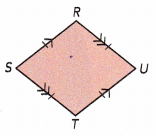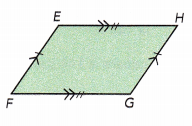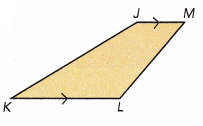# Math in Focus Grade 6 Chapter 10 Answer Key Area of Polygons

Practice the problems of Math in Focus Grade 6 Workbook Answer Key Chapter 10 Area of Polygons to score better marks in the exam.

## Math in Focus Grade 6 Course 1 B Chapter 10 Answer Key Area of Polygons

### Math in Focus Grade 6 Chapter 10 Quick Check Answer Key

Solve.

Question 1.
The length of a rectangle is 15 meters and its width is 8 meters. Find the area of the rectangle.
Given that the length of a rectangle is 15 meters and its width is 8 meters.
Formula to find the area of rectangle will be,
= length × width
Here length = 15m and width = 8m
= 15×8
= 120 sq.m

Solve.

Question 2.
A side length of a square is 10 centimeters. Find the area of the square.
Given that a side length of a square is 10 cm.
Formula to find the area of a square
= length×length
= 10×10
= 100sq.cm
Thus, the area of square will be 100sq.cm

Name each figure and identify the pairs of parallel lines.

Question 3.Given figure RSTU is similar to rhombus.
In rhombus opposite sides are parallel to each other.
Here, line segment RU is parallel to ST and RS is parallel to UT.
Therefore, RS and UT, RU and ST forms the pairs of parallel lines.

Question 4.